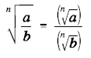#### All Pages

An expression having radicals are in easiest forms when:

• The index cannot be decreased.
• No radicals are in the denominator.

There are four rules of radicals in which will be meaningful in simplifying them.

Rule 1: (n√a)n = n√an = a

Rule 2: n√ab = n√a n√b

Rule 3:Rule 4: n√-a = -n√a, when n is odd.

Examples:

√102  =10

(3√26)3 = 26

√27 = √9.3 = √9 √3 = 3√3

3√-54 = 3√(-27)(2) = (3√-27)(3√2) = -3 3√2

While a radical sign exists in the denominator, it is desirable to erase the radical.  This is done through multiplying both the numerator and denominator through the radical and simplifying.